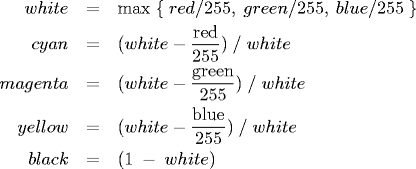COS 126 Conditionals and Loops Programming Assignment

The goal of this assignment is to write five short Java programs to gain practice with expressions, loops and conditionals.

1. Boolean and integer variables and Conditionals. Write a program Ordered.java that reads in three integer command-line arguments, x, y, and z. Define a boolean variable isOrdered whose value is true if the three values are either in strictly ascending order (x < y < z) or in strictly descending order (x > y > z), and false otherwise. Print out the variable isOrdered using System.out.println(isOrdered).
```% java Ordered 10 17 49
true

% java Ordered 49 17 10
true

% java Ordered 10 49 17
false
```

2. Type conversion. There are a number of different formats for representing color. RGB format specifies the level of red (R), green (G), and blue (B) on an integer scale from 0 to 255: It is the primary format for LCD displays, digital cameras, and web pages. CMYK format specifies the level of cyan (C), magenta (M), yellow (Y), and black (K) on a real scale of 0.0 to 1.0: It is the primary format for publishing books and magazines. Write a program RGBtoCMYK.java that reads in three integers red, green, and blue from the command line and prints out equivalent CMYK parameters. The mathematical formulas for converting from RGB to an equivalent CMYK color are:Hint. Math.max(x, y) returns the maximum of x and y.

```% java RGBtoCMYK 75 0 130       // indigo
cyan    = 0.423076923076923
magenta = 1.0
yellow  = 0.0
black   = 0.4901960784313726
```
If all three red, green, and blue values are 0, the resulting color is black (0 0 0 1).

3. Checkerboard. Write a program Checkerboard.java that takes an integer command-line argument N, and uses two nested for loops to print an N-by-N "checkerboard" pattern like the one below: a total of N2 asterisks, where each row has 2N characters (alternating between asterisks and spaces).
 ```% java Checkerboard 4 % java Checkerboard 5 * * * * * * * * * * * * * * * * * * * * * * * * * * * * * * * * * * * * * * * * * ```4. A drunkard's walk. A drunkard begins walking aimlessly, starting at a lamp post. At each time step, the drunkard forgets where he or she is, and takes one step at random, either north, east, south, or west, with probability 25%. How far will the drunkard be from the lamp post after N steps?

1. Write a program RandomWalker.java that takes an integer command-line argument N and simulates the motion of a random walker for N steps. After each step, print the location of the random walker, treating the lamp post as the origin (0, 0). Also, print the square of the final distance from the origin.
```% java RandomWalker 10             % java RandomWalker 20
(0, -1)                           (0, 1)
(0, 0)                            (-1, 1)
(0, 1)                            (-1, 2)
(0, 2)                            (0, 2)
(-1, 2)                           (1, 2)
(-2, 2)                           (1, 3)
(-2, 1)                           (0, 3)
(-1, 1)                           (-1, 3)
(-2, 1)                           (-2, 3)
(-3, 1)                           (-3, 3)
squared distance = 10             (-3, 2)
(-4, 2)
(-4, 1)
(-3, 1)
(-3, 0)
(-4, 0)
(-4, -1)
(-3, -1)
(-3, -2)
(-3, -3)
squared distance = 18
```

2. Write a program RandomWalkers.java that takes two integer command-line arguments N and T. In each of T independent experiments, simulate a random walk of N steps and compute the squared distance. Output the mean squared distance (the average of the T squared distances).
```% java RandomWalkers 100 100000         % java RandomWalkers 400 100000
mean squared distance = 100.15086       mean squared distance = 401.22024

% java RandomWalkers 100 100000         % java RandomWalkers 800 100000
mean squared distance = 99.95274        mean squared distance = 797.5106

% java RandomWalkers 200 100000         % java RandomWalkers 1600 100000
mean squared distance = 199.57664       mean squared distance = 1600.13064
```

As N increases, we expect the random walker to end up further and further away from the origin. But how much further? Use RandomWalkers to formulate a hypothesis as to how the mean squared distance grows as a function of N. Use T = 100,000 trials to get a sufficiently accurate estimate.

Remark: this process is a discrete version of a natural phenomenon known as Brownian motion. It serves as a scientific model for an astonishing range of physical processes from the dispersion of ink flowing in water, to the formation of polymer chains in chemistry, to cascades of neurons firing in the brain.

Submission. Submit the files Ordered.java, RGBtoCMYK.java, Checkerboard.java, RandomWalker.java and RandomWalkers.java. Finally, submit a readme.txt file and answer the questions.